• 第一、 无线信道的多径效应导致的频率选择性衰落 总的来说，这属于“静”，所谓静，就是指发送和接收终端、以及导致电磁波的反射折射等的障碍物都处于静止的状态，而导致多径效应的是这些多种多样的障碍物形成的...
第一、 无线信道的多径效应导致的频率选择性衰落总的来说，这属于“静”，所谓静，就是指发送和接收终端、以及导致电磁波的反射折射等的障碍物都处于静止的状态，而导致多径效应的是这些多种多样的障碍物形成的静态的空间格局。自由空间中是没有多径效应的，有了这些障碍物，同一时刻从发送天线出来电磁波就延不同的方向在不同的时间到达接收天线，在天线上场效应进行叠加而产生了多径分量的混合。换句话说，就是这种复杂多样的空间格局形成了综合的磁波传播环境，这种空间格局具有相应的物理尺寸，对不同频率的电磁波的传播特性是不一样的，所以随着在其中传送的电磁波的频率的变化，其信道响应也不停的变化，这也就是称作频率选择性的本质原因。信道特性随着电磁波频率变化而变化，这种变化延频率轴来看，有快慢、有大小，根据这个原理而定义出信道的对不同频率电磁波的传播特性维持“不变”（就是变化较小）的频率宽度，这个频率宽度称作相干带宽，也就是在此带宽内的电磁波在这个复杂的空间格局中获得近似的传播特性，没有明显的畸变，也就不会导致时域上波形的剧烈变化。相同地，从时域上来看，造成这种波形变化的原因就是不同的传播路径信号的叠加。以直射到达接收端的信号为参考，最迟到达接收端的信号相对直射信号而产生的延后时间T代表了此移动传播环境的多径时延特征，它的倒数正好对应着相干带宽。这就是多经效应的基本原理。第二、 无线信道的多普勒频移导致的时间选择性衰落总的来说，这属于“动”，这个动的意思就是指多普勒频移的产生必须以相对运动为前提，比如接收端相对发送端的运动，反射物相对接收端的运动，反射物相对发送端的运动等等，这些相对运动都会导致接收端接收到的电磁波信号的的频率有所变化。换句话说，因为运动，所以是随着时间而变化的，这也就是称作时变性、时间选择性的一个原因。理论上来讲，如果存在运动，信道的这种时变性就是存在的，只是变化有快慢、有大小。由此而定义出信道的冲激响应维持“不变”的时间间隔的统计平均为此信道的相干时间T。如果一个符号的时间长度短于此相干时间，那么整个符号的波形在传播期间能够得到传播信道较为“一致”的传播，不会发生巨大的畸变。相反则产生时间选择性衰落了。此相干时间的倒数就是最大频偏，其本质意义对应相对运动的速度导致的频率偏移，而相对运动越快（比如一个坐着汽车打手机的人与一个走路打手机的人做比较），传播信道的特性变化自然就越快，对应的相干时间T就越小，那么T的倒数就越大，最大频偏就愈大，与多普勒频移原理完全统一。

总结：  无线信道的时间选择性衰落主要由多普勒平移导致！
无线信道的频率选择性衰落主要由多径效应导致！

大尺度衰落：主要是由于建筑物、高山等的阻挡造成的,因此也叫作阴影衰落.小尺度衰落：接收端收到的信号通常是由发射信号经过多径传输后的矢量合成,多径的随机性使信号的相位也具有随机性,因此接收端信号经过矢量合成后有可能发生严重的衰落.这种衰落往往只要求无线信号经过短时间或短距离传输,我们称之为衰落叫做小尺度衰落,也叫快衰落.
主要由多径效应导致。


展开全文• 路由和负载均衡是P2P计算网格的两个技术难题,由于P2P网络的分布和动态,以及缺乏统一的中心控制,使得传统的路由和负载均衡算法不能应用于P2P网络。提出了一种源自蚁群智能的混合路由和负载均衡算法,通过移动代理,...
• 无线信道由于多径和多普勒频移的原因会在频域出现频率选择性衰落，在时域会出现时间选择性衰落。（在空间会出现空间选择性衰落） 多径引起频率选择性衰落是因为，由于存在多条传播路径，最先到达的信号和最后到达的...
无线信道由于多径和多普勒频移的原因会在频域出现频率选择性衰落，在时域会出现时间选择性衰落。（在空间会出现空间选择性衰落）

多径引起频率选择性衰落是因为，由于存在多条传播路径，最先到达的信号和最后到达的信号之间会有相位差，这个相位差可能是pi，也就是说两个信号可能直接就抵消掉了，这是时域的体现。但是从频域进行分析的时候，因为最长和最短的路径之间距离是一定的，但是频率之间，波长是不一样的，所以他们相差的相位是不一样的。有的频率就会增强（相差2*pi），有的频率就会反向，就造成了有的频率衰落，有的频率增强。所以，体现在频域上就是选择性地衰落。

时间选择性衰落是因为信道的时变，由于接收机或者信道中有移动的物体都会产生时变反射或者时变的信号，所以如果在一个信号的开始和结束，信道发生了剧烈变化，比如由刚开始的h（t）变成了2*h(t)，那信号就会产生巨大变化。可以把信道看成是一个系统，这个系统的冲击响应，如果不变，那我们解码就简单了。如果是时变的，一个符号的时间里面都变了好多次，那解码的时候岂不是凉凉，我们也就得不到原来的信号了。我们把信道的冲激响应基本保持不变的时间叫做相干时间。

空间选择性衰落，我还没找到相关资料，不过我在字面上理解就是，有可能是发射源就是指向性的信号，波束形成之类的；要么就是经过反射多径，导致的空间上的信号的不均匀。比如海面上的雷达，如果冲着海面发射信号，经过海面的反射，最后会形成一个多主瓣的干涉模式。（这段是我自己猜的，暂时还没找到资料）

展开全文• ## 频率选择性衰落、时间选择性衰落

万次阅读 多人点赞 2018-11-08 11:56:26
无线信道的多径效应导致的频率选择性衰落 总的来说，这属于“静”，所谓静，就是指发送和接收终端、以及导致电磁波的反射折射等的障碍物都处于静止的状态，而导致多径效应的是这些多种多样的障碍物形成的静态的空间...
无线信道的多径效应导致的频率选择性衰落

总的来说，这属于“静”，所谓静，就是指发送和接收终端、以及导致电磁波的反射折射等的障碍物都处于静止的状态，而导致多径效应的是这些多种多样的障碍物形成的静态的空间格局。自由空间中是没有多径效应的，有了这些障碍物，同一时刻从发送天线出来电磁波就延不同的方向在不同的时间到达接收天线，在天线上场效应进行叠加而产生了多径分量的混合。换句话说，就是这种复杂多样的空间格局形成了综合的磁波传播环境，这种空间格局具有相应的物理尺寸，对不同频率的电磁波的传播特性是不一样的，所以随着在其中传送的电磁波的频率的变化，其信道响应也不停的变化，这也就是称作频率选择性的本质原因。

信道特性随着电磁波频率变化而变化，这种变化延频率轴来看，有快慢、有大小，根据这个原理而定义出信道的对不同频率电磁波的传播特性维持“不变”（就是变化较小）的频率宽度，这个频率宽度称作相干带宽，也就是在此带宽内的电磁波在这个复杂的空间格局中获得近似的传播特性，没有明显的畸变，也就不会导致时域上波形的剧烈变化。 相同地，从时域上来看，造成这种波形变化的原因就是不同的传播路径信号的叠加。

以直射到达接收端的信号为参考，最迟到达接收端的信号相对直射信号而产生的延后时间T代表了此移动传播环境的多径时延特征，它的倒数正好对应着相干带宽。这就是多经效应的基本原理。

无线信道的多普勒频移导致的时间选择性衰落

总的来说，这属于“动”，这个动的意思就是指多普勒频移的产生必须以相对运动为前提，比如接收端相对发送端的运动，反射物相对接收端的运动，反射物相对发送端的运动等等，这些相对运动都会导致接收端接收到的电磁波信号的的频率有所变化。换句话说，因为运动，所以是随着时间而变化的，这也就是称作时变性、时间选择性的一个原因。

理论上来讲，如果存在运动，信道的这种时变性就是存在的，只是变化有快慢、有大小。由此而定义出信道的冲激响应维持“不变”的时间间隔的统计平均为此信道的相干时间T。如果一个符号的时间长度短于此相干时间，那么整个符号的波形在传播期间能够得到传播信道较为“一致”的传播，不会发生巨大的畸变。相反则产生时间选择性衰落了。此相干时间的倒数就是最大多普勒频偏，其本质意义对应相对运动的速度导致的频率偏移。

信道的时变性是指信道的传递函数是随时间而变化的，即在不同的时刻发送相同的信号，在接收端收到的信号是不相同的。时变性在移动通信系统中的具体体现之一就是多普勒频移（Doppler shift），即单一频率信号经过时变衰落信道之后会呈现为具有一定带宽和频率包络的信号，这又可以称为信道的频率弥散性（frequency dispersion）。

相关系数

对于两个平稳信号S1（t）和S2（t），它们的相关系数的绝对值大于0小于1时，两个信号相关。相关系数等于1时，两个信号相干。当两个信号相干时，它们之间只相差一个复常数。复常数既一有幅度成分，又有频率成分。由此我们可见，若是两个信号相干，它们其中一个可以看作是另一个的幅度的衰减，频率上衰落造成的，其实二者可以看作同一个信号。相关系数越是接近1，相关性越大。

相干时间、相干带宽

相干时间和相干带宽都是描述信道特性的参数。

当两个发射信号的频率间隔小于信道的相干带宽，那么这两个经过信道后的，受到的信道传输函数是相似的。由于通常的发射信号不是单一频率的，即一路信号也是占有一定带宽的，如果，这路信号的带宽小于相干带宽，那么它整个信号受到信道的传输函数是相似的，即信道对信号而言是平坦特性的，非频率选择性衰落的。
同样在相干时间内，两路信号受到的传输函数也是相似的特性，通常发射的一路信号由于多径效应，有多路到达接收机，若这几路信号的时间间隔在相干时间之内，那么他们具有很强的相关性，接收机都可以认为是有用信号，若大于相干时间，则接收机无法识别，只能认为是干扰信号。

ISI

多径效应会引起符号间干扰。详见：https://wenku.baidu.com/view/d0de78c450e79b89680203d8ce2f0066f4336457

总结

多径效应
静

频率选择性衰落

原因：信道特性随着电磁波频率变化而变化，这种变化延频率轴来看，有快慢、有大小

用信道的相干带宽来判断

多普勒效应
动

时间选择性衰落

原因：信道的时变性
用信道的相干时间来判断
相干带宽
定义：信道的对不同频率电磁波的传播特性维持“不变”（就是变化较小）的频率宽度，这个频率宽度称作相干带宽

含义：相干带宽是表征多径信道特性的一个重要参数，它是指某一特定的频率范围，在该频率范围内的任意两个频率分量都具有很强的幅度相关性，即在相干带宽范围内多径信道具有恒定的增益和线性相位。也就是在相干带宽内的电磁波可以获得近似的传播特性。

值：相干带宽等于最大多径时延的倒数（以直射到达接收端的信号为参考，最迟到达接收端的信号相对直射信号而产生的延后时间T代表了此移动传播环境的最大多径时延）

用途：相干带宽用来划分平坦衰落信道和频率选择性衰落信道。从频域看，如果相干带宽小于发送信道的带宽，则该信道特性会导致接收信号波形产生频率选择性衰落，即某些频率成分信号的幅值可以增强，而另外一些频率成分信号的幅值会被削弱。

相干时间
定义：信道的冲激响应维持“不变”的时间间隔的统计平均为此信道的相干时间

含义：相干时间在时域描述信道的频率色散的时变特性。

值:如果信道的最大多普勒频移为fm，那么信道的相干时间Tc=0.423/fm；也有说相干时间是多普勒频移的倒数，Tc=1/fm

用途：相干时间用来划分快衰落（时间选择性衰落）和慢衰落（时间非选择性衰落）。如果基带信号的符号周期大于信道的相干时间，则在基带信号的传输过程中信道可能会发生改变，导致接收信号发生失真，产生时间选择性衰落，也称快衰落；如果基带信号的符号周期小于信道的相干时间，则在基带信号的传输过程中信道不会发生改变，也不会产生时间选择性衰落，也称慢衰落。

展开全文相干时间
• 讨论思路信道容量定义及物理意义定义物理意义（David 无线通信基础）AWGN信道信道容量AWGN信道（离散时间信道容量：平坦衰落信道的容量CDI 已知CSIR各态历经容量内容与AWGN容量的关系使用场景中断容量内容适用...
无线信道的信道容量总论来源：讨论思路信道容量定义及物理意义定义物理意义（David 无线通信基础）AWGN信道下信道容量AWGN信道（离散时间）信道容量：平坦衰落信道的容量CDI 已知CSIR各态历经容量内容与AWGN容量的关系使用场景中断容量内容适用场景CSIRT系统模型功率控制最优功率控制（注水法）求解物理意义次优功率控制（信道反转）各容量对比频率选择性信道时不变时变
总论
来源：

Andrea Goldsmith-Wireless Communication
David Tse-Fundamentals of Wireless Communication

讨论思路
首先从定义和物理意义角度讨论无线信道的容量是什么，此处内容主要来自于 David Tse-Fundamentals of Wireless Communication。
之后讨论在不同信道以及不同通信场景下，信道容量的值，主要有两个维度：

不同信道（AWGN信道、平稳时变信道、频率选择性时不变以及时变信道）
不同通信场景（收端知信道分布信息CDI、收端知信道信息CSIR、收发都知CSIRT）

信道容量定义及物理意义
定义
1、当误码率接近无限小时，通信系统所允许的最高传输速率。
$C=max(R_{\mathrm{b}}|BER \rightarrow 0)$
2、信道输入与输出的互信息量的最大值。
$C=\text{max }I(\text{input;output})$
物理意义（David 无线通信基础）
根据采样定理，任何一个带宽为$B$，时长为$T$的信号，至少需要$N=2BT$个样本才能重建，所以信号空间为$N$维，所以任意一个信号可以看成是$N$维欧氏空间中的一个点。
假设给定发送信号功率约束$P$,高斯白噪声的方差为$\sigma^{2}$，根据大数定律，$N$维接受矢量将高概位于半径为$\sqrt{N{P+\sigma^2}}$的超球内，而考虑噪声的影响，对于发射星座点，对应接受矢量应高概位于半径为$\sqrt{N}\sigma$的噪声球附近。如果要无误通信，相邻噪声球不应该重叠，所以物理意义是将多个噪声球填充进入$N$维信号空间的超球中，最大填充个数则是我们的信道容量：
$\frac{\sqrt{N\left(P+\sigma^{2}\right)}^{N}}{\sqrt{N \sigma^{2}} N}$
比特化后有：
$\frac{1}{T} \log \left(\frac{\sqrt{N\left(P+\sigma^{2}\right)}^{N}}{\sqrt{N \sigma^{2}} N}\right)=B \log \left(1+\frac{P}{\sigma^{2}}\right)$
物理意义图示如下：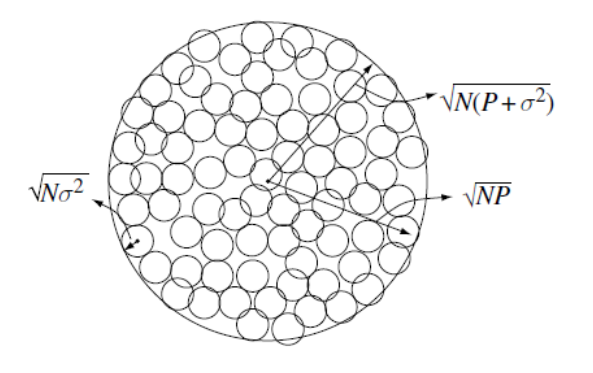此处推荐知乎回答
AWGN信道下信道容量
AWGN信道（离散时间）
$y[i]=x[i]+n[i]$
AWGN信道无衰落，所以接受信噪比是恒定值$\gamma=P /\left(N_{0} B\right)$
信道容量：
$C=B \log _{2}(1+\gamma)$
容量最大输入是高斯分布。
香农容量是数据率的上界。
平坦衰落信道的容量
在AWGN基础上加入了平坦衰落，时变的信道增益系数为$\sqrt{g[i]}, 0 \leq g[i]$，系统模型为：
$y[i]=\sqrt{g[i]} x[i]+n[i]$
系统框图：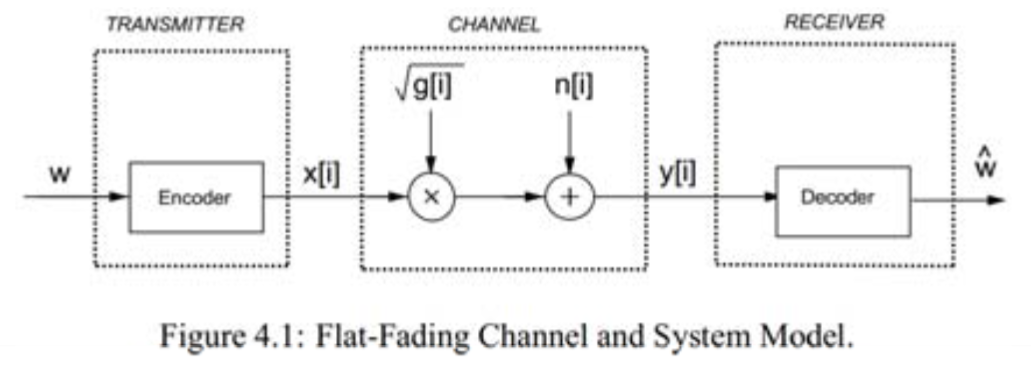信道功率增益系数$g[i]$服从分布$p(g)$，则接受信噪比为：
$\gamma[i]=\bar{P} g[i] /\left(N_{0} B\right), 0 \leq \gamma[i]<\infty$
其中$\bar{P}$为平均发送功率。
对于信道增益的先验信息有三种：

信道分布信息(CDI)已知——收发都已知 $g[i]$的分布；
接收端已知(CSIR)——接收端已知$g[i]$在时刻$i$的值，且收发都已知$g[i]$的分布；
发送端和接收端都已知(CSITR)。

CDI 已知
容量求解，用下式：
$C=\max _{p(x)} I(X ; Y)=\max _{p(x)} \sum_{x, y} p(x, y) \log \left(\frac{p(x, y)}{p(x) p(y)}\right)$
求解困难
CSIR
此时有两种讨论容量的角度：

各态历经容量：平均误码率为零的最大传输速率。
中断容量：在某个中断概率下的信道最大传输速率。

各态历经容量
内容
$C=\int_{0}^{\infty} B \log _{2}(1+\gamma) p(\gamma) d \gamma$
这是一个理论恒定值，即不可将其理解为，当接受信噪比为$\gamma$时，速率为$B \log _{2}(1+\gamma)$，因为发端没有CSI，只能以恒定速率传输
与AWGN容量的关系
\begin{aligned} &\begin{aligned} E\left[B \log _{2}(1+\gamma)\right] &=\int B \log _{2}(1+\gamma) p(\gamma) d \gamma \\ & \leq B \log _{2}(1+E[\gamma])\\ &=B \log _{2}(1+\bar{\gamma}) \end{aligned}\\ \end{aligned}
可见对于相同的平均接受信噪比$\bar{\gamma}$，CSIR的信道容量小于AWGN的信道容量，这说明信道衰落总是会降低容量。
使用场景
快变信道，因为其要求信道编码应该遍历各个信道状态，而慢变信道造成的编码长度太长。
中断容量
在允许一定误码时（出错重传）的信道容量，则与各态历经相比则可以获得更高的信道容量。
内容
由可接受中断概率$p_{out}$给一个最小的接受信噪比$p_{\text {out }}=p\left(\gamma<\gamma_{\min }\right)$，则中断容量为：
$C=B \log _{2}\left(1+\gamma_{\min }\right)$
平均正确接收的信息率为：
$C_{o}=\left(1-p_{\text {out }}\right) B \log _{2}\left(1+\gamma_{\min }\right)$
适用场景
慢变信道
CSIRT
系统模型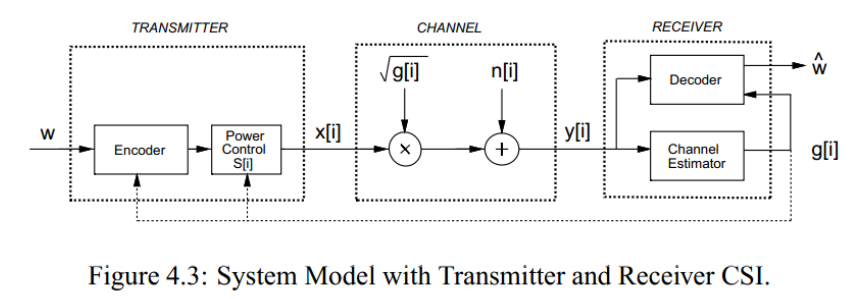CSIT引入最大的不同在于，发端可以控制信息传输速率以及功率控制，进而提供容量增益。
此时信道容量为：
$C=\int_{0}^{\infty} C_{\gamma} p(\gamma) d \gamma=\int_{0}^{\infty} B \log _{2}(1+\gamma) p(\gamma) d \gamma$
从上式可以看出，当CSIRT时，如果不进行功率控制，仅根据接收信噪比调整发送速率，不会增加信道容量，但可以有效提高系统的平均传输速率（使其接近CSIR信道容量）
功率控制
进行功率分配的过程数学化为：
$C=\max _{P(\gamma): \int P(\gamma) p(\gamma) d \gamma=\bar{P}} \int_{0}^{\infty} B \log _{2}\left(1+\frac{P(\gamma) \gamma}{\bar{P}}\right) p(\gamma) d \gamma$
最优功率控制（注水法）
求解
对上式，构造Lagrange：
$J(P(\gamma))=\int_{0}^{\infty} B \log _{2}\left(1+\frac{\gamma P(\gamma)}{\bar{P}}\right) p(\gamma) d \gamma-\lambda \int_{0}^{\infty} P(\gamma) p(\gamma) d \gamma$
令导数为零求极值：
$\frac{\partial J(P(\gamma))}{\partial P(\gamma)}=\left[\left(\frac{B / \ln (2)}{1+\gamma P(\gamma) / \bar{P}}\right) \frac{\gamma}{\bar{P}}-\lambda\right] p(\gamma)=0$
解得最优功控：
$\frac{P(\gamma)}{\bar{P}}=\left\{\begin{array}{ll} \frac{1}{\gamma_{0}}-\frac{1}{\gamma} & \gamma \geq \gamma_{0} \\ 0 & \gamma<\gamma_{0} \end{array}\right.$
其中$\gamma_{0}$为中断门限，表明我们仅在$\gamma_{0} \leq \gamma[i]<\infty$使用信道。且满足：
$\int_{\gamma_{0}}^{\infty}\left(\frac{1}{\gamma_{0}}-\frac{1}{\gamma}\right) p(\gamma) d \gamma=1$
$\gamma_{0}$无闭式解，通过数值解法求解。
将上式代入原信道容量公式，有：
$C=\int_{\gamma_{0}}^{\infty} B \log _{2}\left(\frac{\gamma}{\gamma_{0}}\right) p(\gamma) d \gamma$
物理意义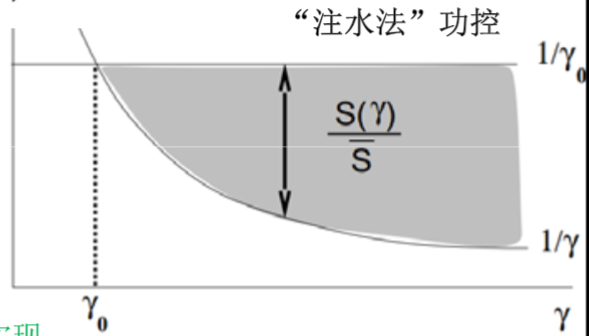$1/\gamma_{0}$为水深，$1/\gamma$为碗深，我们向碗中注水直至水的总体积为总功率。
表明，在数据传输过程中，我们在信道条件好的时候（$\gamma$较大时），多分配功率，且用高速率传输；在信道条件差的时候（$\gamma$较小时），少分配功率，且用低速率传输；如果信道条件太差（低于$\gamma_{0}$），则不进行数据传输。
次优功率控制（信道反转）
恒速率传输，用功控直接抵消信道衰减的影响，使得整个信道等效于AWGN信道。
信道反转由$P(\gamma) / \bar{P}=\sigma / \gamma$确定，$\sigma$是满足功率约束条件下的恒定接收信噪比。
零中断信道容量为：
$C=B \log _{2}[1+\sigma]=B \log _{2}\left[1+\frac{1}{\mathbf{E}[1 / \gamma]}\right]$
上述也是零中断信道反转容量，当考虑中断概率时，只有条件好于中断门限才补偿，否则不传：
$\frac{P(\gamma)}{\bar{P}}=\left\{\begin{array}{ll} \frac{\sigma}{\gamma} & \gamma \geq \gamma_{0} \\ 0 & \gamma<\gamma_{0} \end{array}\right.$
则中断概率为$p_{out}$时吞吐量为：
$C\left(p_{\text {out }}\right)=B \log _{2}\left(1+\frac{1}{\mathbf{E}_{\gamma_{0}}[1 / \gamma]}\right) p\left(\gamma \geq \gamma_{0}\right)$
取中断概率使得上式最大即为最大中断容量：
$C=\max _{\gamma_{0}} B \log _{2}\left(1+\frac{1}{\mathbf{E}_{\gamma_{0}}[1 / \gamma]}\right) p\left(\gamma \geq \gamma_{0}\right)$
其中$\mathbf{E}_{\gamma_{0}}[1 / \gamma] \triangleq \int_{\gamma_{0}}^{\infty} \frac{1}{\gamma} p(\gamma) d \gamma$
各容量对比
下面有对数正态分布，瑞利衰落、Nakagami衰落下容量对比，三种信道衰落剧烈程度递减：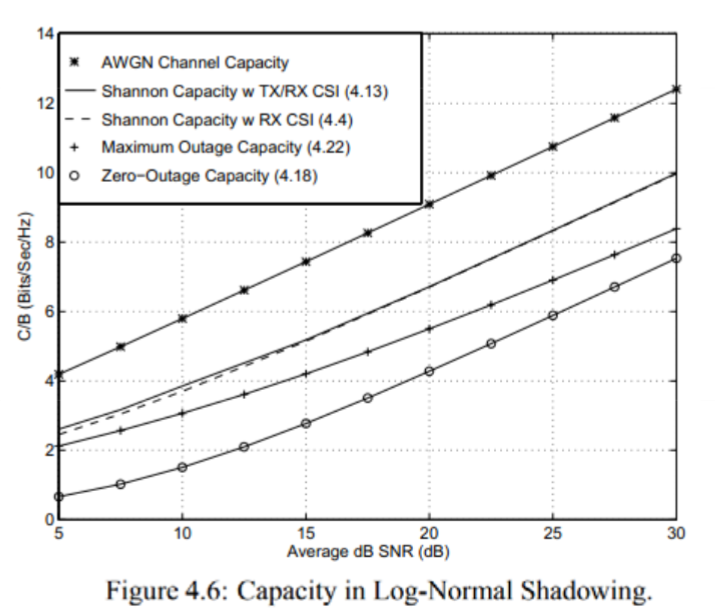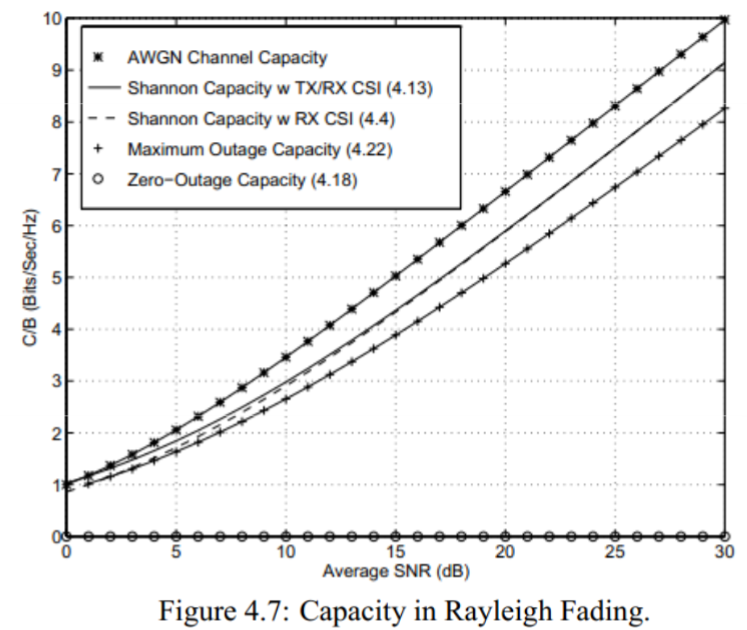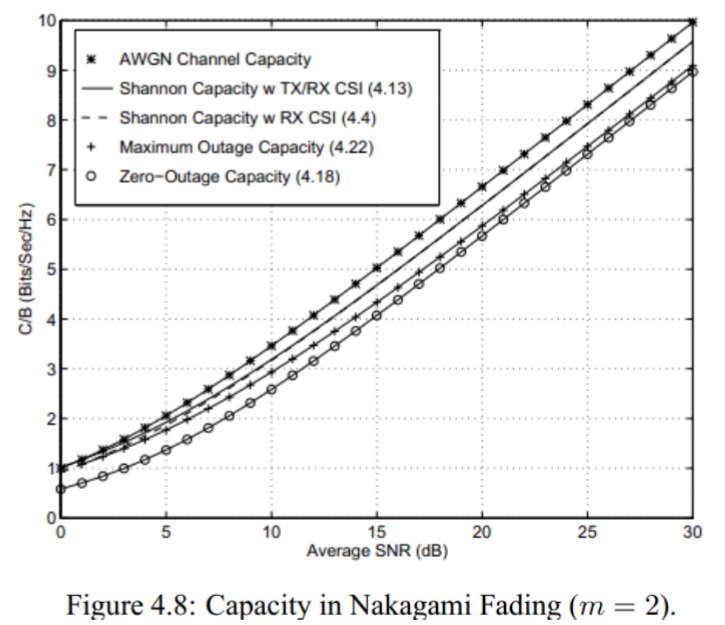如图关注三个问题：

随着信道衰减变得不剧烈（图1->图3），可以看到各个容量逐渐靠近与AWGN信道，且AWGN为任何情况最大容量。
瑞利衰落及Nakagami衰落可以看出，低信噪比下CSIRT接近AWGN甚至大于AWGN，这是由于AWGN是高信噪比下的恒速率传输，当平均信噪比低时，其容量较低，而CSIRT由于是注水法功控，放弃了低信噪比信道而集中功率及速率与偶尔出现的高信噪比信道实现，则会出现容量高于AWGN的情况。
CSIRT相比CSIR，比较靠近且增益不大，这说明信道容量增益主要贡献者是发端的速率控制，功率控制的贡献度不大。

频率选择性信道
基本等同于平台衰落，仅是问题构建不同，这里只讨论CSIRT
时不变
系统框图：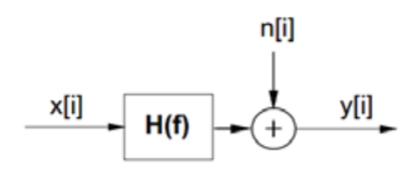按频率切开：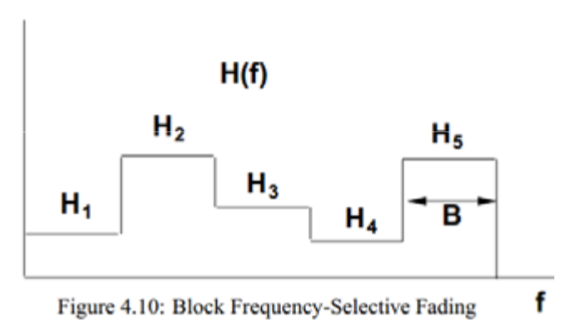容量：
$C=\sum_{\max P_{j}: \sum_{j} P_{j} \leq P} B \log _{2}\left(1+\frac{\left|H_{j}\right|^{2} P_{j}}{N_{0} B}\right)$
功控采用频率注水：
$\frac{P_{j}}{P}=\left\{\begin{array}{ll} \frac{1}{\gamma_{0}}-\frac{1}{\gamma_{j}} & \gamma_{j} \geq \gamma_{0} \\ 0 & \gamma_{j}<\gamma_{0} \end{array}\right.$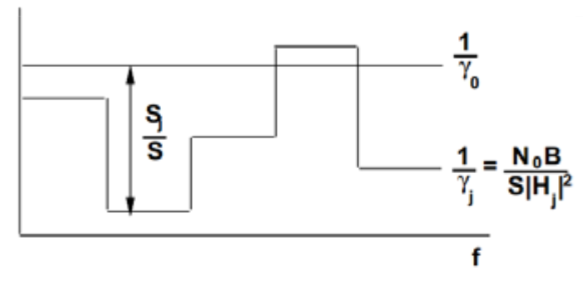时变
先在频率上用相关带宽切开，频率之间用注水，得到的每一块在时间上用注水（二维注水）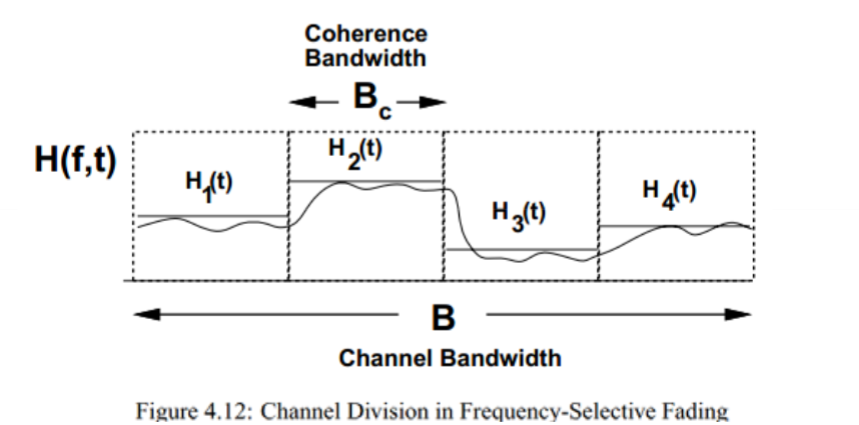展开全文• ## MIMO信道的信道容量

千次阅读 2015-12-29 15:47:08
假设信道容量分析模型的信道的衰落类型是频率非选择性衰落，信道系数为0均值单位方差的复高斯随机变量。假设系统的发射端配有NT根天线，接收端配有NR根天线，总的发射功率为P。假设发射端未知信道的瞬时状态信息，...
• 认知无线电采用无线电域的基于模型的方法对控制无线电频谱使用的规则（如射频频段、空中接口、协议以及空间和时间模式等）进行推理，通过无线电知识表示语言（RKRL），表述无线电规则、设备、软件模块、电波传播特性...
• ## 平坦衰落信道建模

千次阅读 2013-10-29 20:55:36
根据信道的时间选择性，可以将信道分为块衰落信道和慢衰落信道；根据信道的空间选择特性可以将信道分为标量信道和矢量信道。由于平坦衰落只有一个可分辨径（包括了多条不可分辨径），而频率选择性信道是由多个具有...
• T3题：信道安全 时间限制：1000 ms | 内存限制：65535 KB 描述 Alpha 机构有自己的一套网络...经过测试分析，Alpha 情报系统获得了网络中每段信道安全可靠的概率，情报员 A 决定选择一条安全最高，即概率最大的最短路径
• 认知无线电采用无线电域的基于模型的方法对控制无线电频谱使用的规则（如射频频段、空中接口、协议以及空间和时间模式等）进行推理，通过无线电知识表示语言（RKRL），表述无线电规则、设备、软件模块、电波传播特性...
• 窄带：信号带宽小于多径信道的相关带宽。 宽带：信道带宽大于多径信道的相关带宽。 相干带宽是表征多径信道特性的一个重要参数，它是指某一特定的频率范围，在该频率范围内的任意两个频率分量都具有很强的幅度相关性...
• 在学习瑞利衰落信道时整理的资料，包括了平坦瑞利衰落和频率选择性衰落的介绍书籍，移动衰落信道、MIMO-OFDM无线通信仿真等书籍，以及改进的瑞利衰落信道资料，介绍瑞利衰落信道相关带宽、相关时间等基本概念的文档...
• 代码分别对多径多普勒信道进行了时域和频域的分析，通过观测时间的不同展现了信道时间选择性衰落和频率选择性衰落，适合初学者理解
• ## 无线多径信道

千次阅读 2019-03-18 15:35:02
相干时间 时延扩展–相干带宽，频率选择或者频率平坦衰落 信道时变？ 相干时间和多普勒扩展成反比 CT=1fmCT = \frac{1}{fm}CT=fm1​ ...频率选择性衰落fc&lt;W 频率选择性慢衰落 频率选择性快衰落 人...
• 信道衰落包括快衰落和慢衰落。引起两方面的作用：降低了信号的强度，更... 时间选择性衰落：信道在不同的时间的衰落不一样。相干时间（信道保持不变的时间）和多普勒频移成反比。 频率选择性衰落：在不同频率...通信技术
• 信道时间色散的:在频域,幅频响应呈频率选择性衰落,相频响应基本上为线性相位;在时域,信道的冲激响应表现出时延扩展。rms时延扩展和相干带宽均与具体的信道结构有关,大致在数十微秒和数十千赫兹数量级。信道的窄带...电力线通信
• 如果信号的带宽远大于信道的相干带宽，那么会引起频率选择性衰落(时域上等价于信号的码字持续时间小于信道的时延扩展，就会引起码间串扰)。为了避免信号严重失真，一般要求传输信号的带宽要小于相干带宽，这种衰...
• 高速移动下的无线宽带通信要经历时间和频率的双选择性衰落,为了使发送的数据经过衰落的信道后在接收端被正确地接收,必须要对信道状态信息进行估计。本文根据双选信道在时延-多普勒域具有稀疏性,研究了OFDM系统中基于...
• 协作分集技术解决了MIMO技术在移动终端受天线制约的问题由于无线通信环境的特殊性和复杂性，对信道估计十分困难，因此引入了不需要信道估计的差分空时编码，并在时间选择性衰落信道和频率选择性衰落信道的基础上...
• ## 信道估计系列之LS

千次阅读 2019-09-21 04:33:16
在无线通信系统中，系统的性能主要受到无线信道的制约。基站和接收机之间的传播路径复杂多变，从简单的...而由于时间选择性衰落和频率偏移带来的子载波干扰（ICI），除了依靠时频偏补偿来纠正外，还需要对信道进行...
• 用LP标准，使路由选择标准在链路生存时间和最短路径之间取得均衡，这样选择信道在移动环境中更加具有可靠；用基于距离的信道选择算法选择信道，该信道选择算法首先估算节点间距离，根据估算到的距离选择合适的...
• 该程序可生成时间总长度为1min的线性调频信号或单频调制信号，分别用于测试水声信道的多途时延结构和信道频率选择性衰落。
• 在高速移动性场景下，无线信道呈现频率-时间选择性，若要实现信道估计，则需引入大量导频。针对上述问题，提出一种结合分布式压缩感知理论的信道估计导频优化方案。首先，根据时延域中无线信道的稀疏特性挖掘基...分布式压缩感知
• 针对多信道感知无线电网络中现有协作式频谱感知方法的不足,考虑信道使用特征、检测性能的动态以及SU选择感知信道时需对PU造成的干扰降至最低等因素,将信道分配问题建模为非线性整数规划问题.为高效地获得该问题的...
• 基于序贯蒙特卡罗算法的MIMO信道跟踪，阙大顺，刘宇，针对无线通信信道具有时间选择性与频率选择性两个特征，目前流行的空时处理技术的关键假设在于接收机已知MIMO信道状态信息，而MIMO�
• 信道衰落系数越大、编码速率越大、收发天线数目越多，通信系统的RCEE越大，相应的译码错误概率越小，系统的通信可靠越高。对于给定译码错误概率的MIMO无线通信系统，可以通过计算系统的RCEE来估计信道所需的编码...
• 但是，由于随时间变化的信道衰落和移动卫星系统中巨大的往返延迟，自适应方法的应用受到很大限制。 为了解决这个问题，本文提出了一种基于移动卫星链路部分信道信息的ACM方案，并推导了一种优化的自适应策略和功率...研究论文
• 发射机与接收机之间的相对运动会产生多普勒频移。 各多径分量经历了不同的多普勒频移...若信道的所有多径分量的传播时延τ\tauτ远小于Ts，则称为平坦衰落信道（频率非选择性衰落信道）。 在通带范围内，信道特性是...信号处理...